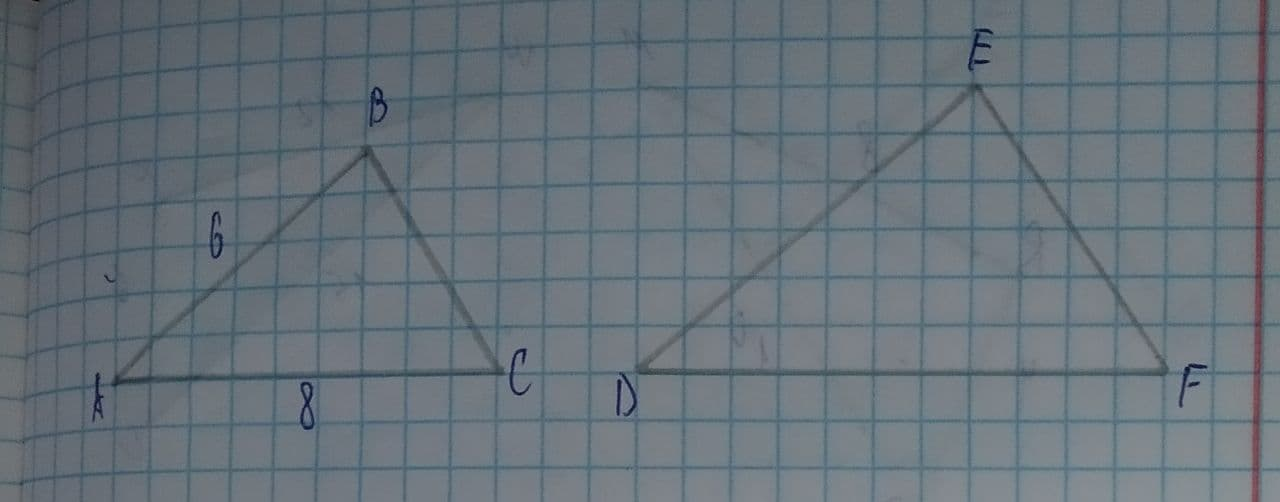# \triangle ABC\sim \triangle DEF If AB=6 and AC=8, which statement will justify similarity by SAS?ddaeeric 2021-07-30 Answered
In the diagram, $\mathrm{△}ABC\sim \mathrm{△}DEF$.If AB=6 and AC=8, which statement will justify similarity by SAS?
1) DE=9, DF=12, and $\mathrm{\angle }A\stackrel{\sim }{=}\mathrm{\angle }D$
2) DE=8, DF=10, and $\mathrm{\angle }A\stackrel{\sim }{=}\mathrm{\angle }D$
3) DE=36, DF=64, and $\mathrm{\angle }C\stackrel{\sim }{=}\mathrm{\angle }F$
4) DE=15, DF=20, and $\mathrm{\angle }C\stackrel{\sim }{=}\mathrm{\angle }F$
You can still ask an expert for help

• Questions are typically answered in as fast as 30 minutes

Solve your problem for the price of one coffee

• Math expert for every subject
• Pay only if we can solve itAdnaan Franks
Step 1
Given Data:
The length of side AB is: AB=6
The length of side AC is: AC=8
According to SAS, two pairs of corresponding sides of both triangles should be in the same proportion, and the angle made by these two adjacent lines of each triangle is congruent then they call similar triangle by SAS similarity.
From SAS similarity rule,
Condition 1: $\frac{AB}{DE}=\frac{AC}{DF}$
Condition 2: $\mathrm{\angle }A\stackrel{\sim }{=}\mathrm{\angle }D$
Step 2
For option (1), DE=9, DF=12, $\mathrm{\angle }A\stackrel{\sim }{=}\mathrm{\angle }D$
Substitute all the values in condition 1,
$\frac{6}{9}=\frac{8}{12}$
0.67=0.67
Option (1) satisfies condition 2 too.
So, option (1) satisfies each condition.
For option (2), DE=8, DF=10, $\mathrm{\angle }A\stackrel{\sim }{=}\mathrm{\angle }D$
Substitute all the values in condition 1,
$\frac{6}{8}=\frac{8}{10}$
$0.75\ne q0.8$
So, option (2) satisfies only condition 2.
Step 3
For option (3), DE=36, DF=64, $\mathrm{\angle }C\stackrel{\sim }{=}\mathrm{\angle }F$
Substitute all the values in condition 1,
$\frac{6}{36}=\frac{8}{64}$
$0.17\ne q0.125$
Option (3) does not satisfy condition 2.
For option (4), DE=15, DF=20, $\mathrm{\angle }C\stackrel{\sim }{=}\mathrm{\angle }F$
Substitute all the values in condition 1,
$\frac{6}{15}=\frac{8}{20}$
0.4=0.4
Option (4) does not satisfy condition 2.
Thus, option (1) is the correct option.